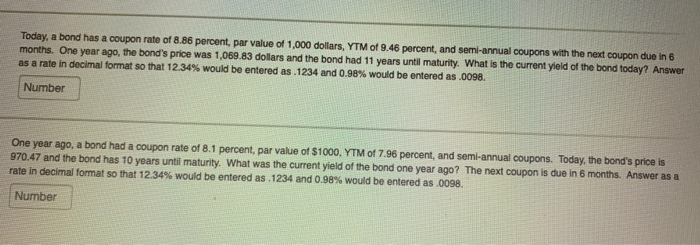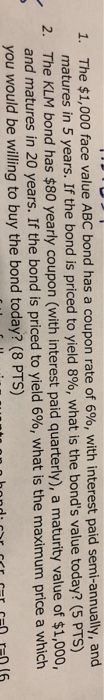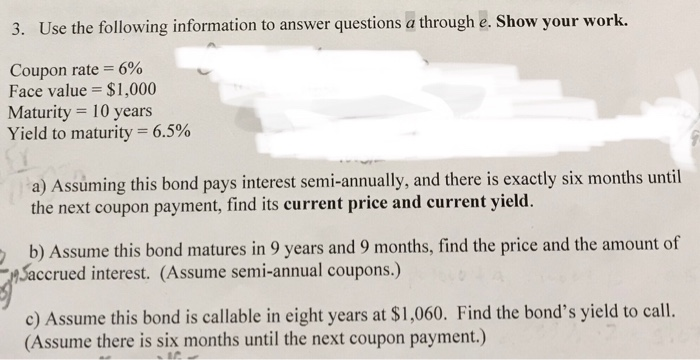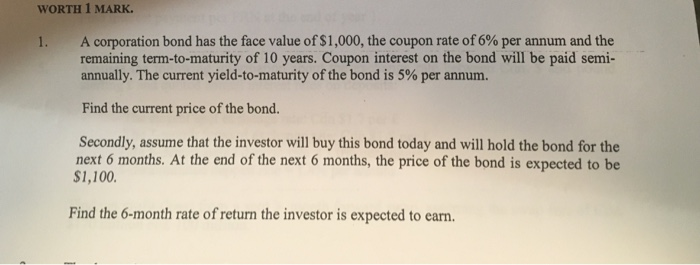Question

1) Consider a 10-year bond trading at \$1150 today. The bond has a face value of \$1,000, and has a coupon rate of 8%. Coupons are paid semiannually, and the next coupon payment is exactly 6 months from now. What is the bond's yield to maturity?

2)A coupon-paying bond is trading below par. How does the bond's YTM compare to its coupon rate?

b. YTM = Coupon Rate

c. YTM > Coupon Rate

d. YTM < Coupon Rate

3)A coupon-paying bond is trading at par. How does the bond's current yield compare to its coupon rate?

b. YTM = Coupon Rate

c. YTM > Coupon Rate

d. YTM < Coupon Rate

4) Consider a bond with a coupon rate of 6% and a face value of \$1,000. Coupons are paid semi-annually. Suppose there are 94 days to the next coupon payment date. Assuming a 30/360 day-count convention, what is the accrued interest on this bond today?

5) Consider a bond with a coupon rate of 7% and a face value of \$1,000. Coupons are paid semi-annually. Suppose there are 52 days to the next coupon payment date, beyond which there are 2 years left to maturity (so that there are in total 1+2*2 number of coupon payments left). The bond is currently trading at a YTM of 4%. Assuming a 30/360 day-count convention, what is the bond's full (dirty) price?

6) When buying a bond, an investor pays the clean (or the quoted) price.

a.True

b. False

7)Coupon-bearing Treasury securities pay coupons semi-annually.

a. True

b.False

8)

Calculate the full (dirty) price of a bond, assuming the following.

Transaction settlement date is July 11th, 2018.

Bond maturity date is September 30th, 2020.

Coupon rate is 5%, and coupons are paid semi-annually.

The bond is trading at a YTM of 8%.

The day-count convention is 30/360.

Redemption value is 100.

9)

Consider a corporate bond with a face value of \$1,000, 2 years to maturity and a coupon rate of 6%. Coupons are paid semi-annually. The next coupon payment is to be made exactly 6 months from today. What is this bond's price assuming the following spot rate curve.

6-month spot rate: 3%.

12-month: 5%.

18-month: 5.5%.

24-month: 5.8%.

10)

Consider a corporate bond with a face value of \$1,000, 2 years to maturity and a coupon rate of 5%. Coupons are paid semi-annually. The next coupon payment is to be made exactly 6 months from today. What is this bond's YTM assuming the following spot rate curve.

6-month spot rate: 4%.

12-month: 5%.

18-month: 5.5%.

24-month: 5.8%.

11) What is the current yield of a 6-year Treasury note with a coupon rate of 5%, a face value of \$100, and is currently trading at 100:10?

Yeild to maturity (YTM) can be calculated using two methods:

a) Approximation Method

b) IRR Method

Both the methods gives approximately same results.

we are using approximation method to solve this question.

YTM= (C+(F-P)/n) / ((F+P)/2)

where C= Coupon Payment/Interest Payment

F= Face Value

P=Price

n=years to maturity

Putting Values in the equation above:

YTM= (1000*4%+(1000-1150)/20) / ((1000+1150)/2)

Coupon rate is 8% for an year; so 4% semi annually

years to maturity is 10 years; 20 semi annual

YTM=(40+(-7.5))/1075

YTM=3.02% semi annually

So YTM= 3.02%*2= 6.05% annually

If a coupon paying bond is trading below par ; lets say 999

putting the above price in above formulla

YTM= (1000*4%+(1000-999)/20)/ ((1000+999)/2)

YTM= 4.01% semi annually

YTM= 8.01% annually

So correct answer is (C) YTM> Coupon Rate

If a bond is trading at par; i.e 1000

YTM=(1000*4%+(1000-1000)/20)/((1000+1000)/2)

YTM=4 % semi annually

YTM= 8% annually

SO correct part is (b) YTM=Coupon Rate

coupon rate= 6% annually , so 3% semi annually

Assumed 360 days in an year, so 180 days in 6 months

94 days left for the next coupon payment, it means we have already earned interest for 86 days (180 days-94 days)

Accrued Interest= 1000*3%*86/180

Accried Intererst = \$14.33

#### Earn Coins

Coins can be redeemed for fabulous gifts.

Similar Homework Help Questions
• ### 25-year bond has a \$1,000 face value, a 10% yield to maturity, and an 8% annual...

25-year bond has a \$1,000 face value, a 10% yield to maturity, and an 8% annual coupon rate, paid semi-annually. What is the market value of the bond? Suppose a bond with a 10% coupon rate and semiannual coupons, has a face value of \$1000, 20 years to maturity and is selling for \$1197.93. What’s the YTM?

• ### Today, a bond has a coupon rate of 8.86 percent, par value of 1,000 dollars, YTM...Today, a bond has a coupon rate of 8.86 percent, par value of 1,000 dollars, YTM of 9.46 percent, and semi-annual coupons with the next coupon due in 6 months. One year ago, the bond's price was 1,069.83 dollars and the bond had 11 years until maturity. What is the current yield of the bond today? Answer as a rate in decimal format so that 12.34% would be entered as.1234 and 0.98% would be entered as .0098. Number One year...

• ### Springfield Nuclear Energy Inc. bonds are currently trading at \$1291.39, The bonds have a face value...

Springfield Nuclear Energy Inc. bonds are currently trading at \$1291.39, The bonds have a face value of \$1,000 a coupon rate of 10.5% with coupons paid annually, and they mature in 15years. What is the yield to maturity of the bonds? The yield to maturity of the bonds is ____ beam inc. bonds are trading today for a price of ​\$798.96. the bond pays annual coupons with a coupon rate of 6​% and the next coupon is due in one...

• ### A bond has a quoted price of \$900 today. It has a face value of \$1,000,...

A bond has a quoted price of \$900 today. It has a face value of \$1,000, coupon rate of 12%, and a time to maturity of 6 years. Coupons are paid every month. What is its yield to maturity?

• ### A fixed coupon bond has a coupon rate of 4% paid semi-annually with a maturity date...

A fixed coupon bond has a coupon rate of 4% paid semi-annually with a maturity date of 8/15/20. The bond uses a 30/360 day counting convention and is trading at a yield today (12/10/19) of 3%. Calculate the following for today: The Dirty Price of the Bond

• ### formula + answer please 1. The \$1,000 face value ABC bond has a coupon rate of...formula + answer please 1. The \$1,000 face value ABC bond has a coupon rate of 6%, with interest paid semi-annually, and matures in 5 years. If the bond is priced to yield 8%, what is the bond's value today? (5 PTS) 2. The KLM bond has \$80 yearly coupon (with interest paid quarterly), a maturity value of \$1,000, and matures in 20 years. If the bond is priced to yield 6%, what is the maximum price a which you...

• ### ouphi& Shour ur wort. Coupon rate 6% Face value \$1,000 Maturity 10 years Yield to maturity-6.5%...ouphi& Shour ur wort. Coupon rate 6% Face value \$1,000 Maturity 10 years Yield to maturity-6.5% a) Assuming this bond pays interest semi-annually, and there is exactly six months until the next coupon payment, find its current price and current yield. b) Assume this bond matures in 9 years and 9 months, find the price and the amount of Saccrued interest. (Assume semi-annual coupons.) c) Assume this bond is callable in eight years at \$1,060. Find the bond's yield to...

• ### A fixed coupon bond has a coupon rate of 4% paid semi-annually with a maturity date...

A fixed coupon bond has a coupon rate of 4% paid semi-annually with a maturity date of 8/15/20. The bond uses a 30/360 day counting convention and is trading at a yield today (12/10/19) of 3%. Calculate the following for today: The Dirty Price of the Bond Answer this: The Accrued Interest of the Bond

• ### A fixed coupon bond has a coupon rate of 4% paid semi-annually with a maturity date...

A fixed coupon bond has a coupon rate of 4% paid semi-annually with a maturity date of 8/15/20. The bond uses a 30/360 day counting convention and is trading at a yield today (12/10/19) of 3%. Calculate the following for today: The Dirty Price of the Bond The Accrued Interest of the Bond Answer this :The Clean Price of the Bond

• ### WORTH 1 MARK 1. A corporation bond has the face value of \$1,000, the coupon rate...WORTH 1 MARK 1. A corporation bond has the face value of \$1,000, the coupon rate of 6% per annum and the remaining term-to-maturity of 10 years. Coupon interest on the bond will be paid semi- annually. The current yield-to-maturity of the bond is 5% per annum. Find the current price of the bond. Secondly, assume that the investor will buy this bond today and will hold the bond for the next 6 months. At the end of the next...